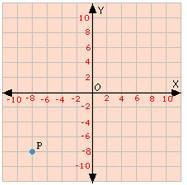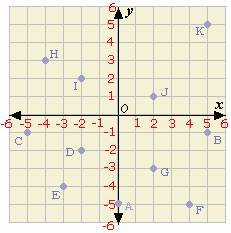## Definition Of Coordinates

In a two-dimensional coordinate plane, Coordinates are the pairs of numbers which specify the position or location of a point or of an object.

Coordinates are represented by putting the ordered pairs in parentheses. For example: (x, y).
X-coordinate is the first number in an ordered pair and represents the horizontal position of an object in the coordinate plane.
Y-coordinate is the second number in an ordered pair and represents the vertical position of an object in the coordinate plane.

### Example of CoordinatesThe point P is eight units to the left of the Y-axis (negative direction) and is eight units below the X-axis (negative direction). So the coordinates of point P is (- 8, - 8).

### Solved Example on Coordinates

#### Ques: Identify the coordinates of the point C from the graph.A. (- 5, 1)
B. (5, - 1)
C. (- 1, - 5)
D. (- 5, - 1)